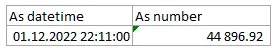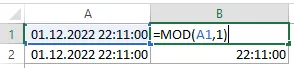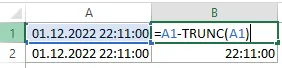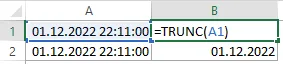# Extract time from DateTime in Excel

The fastest way to extract time in Excel is by using the function MOD. The function MOD in Excel returns the remainder after a number divided by a divisor. In other words, you can extract decimals. The time part in DateTime is decimal numbers; by using MOD, you can get only the fractional part. By using this trick, you can get only time from DateTime in Excel.

## Extract time from DateTime in Excel

Here is my date and time combination. At the same time, if it is formatted as a number, you can see that time is represented as decimals.22 hours and 11 minutes is about 92% of the whole day.

With function MOD you can divide the date and time by one (nothing changes) and return a reminder (time).

`=MOD(A1,1)`There might be necessary to adjust the format if the result contains the date and time like this.

`00.01.1900  22:11:00`

If you want to get a specific time format in Excel, try to use format code. Here are great examples of what you can do with that to format Excel chart axis values conditionally or as thousands.

Alternatively, you can get time from DateTime in Excel by using the function TRUNC that removes the fractional part of the number, like in this example. Mathematically you can get the same result as previously.

`=A1-TRUNC(A1)`## Date from date and time in Excel

If the Excel function TRUNC removes decimals, you can also use that to get the date part from date and time.

`=TRUNC(A1)`